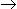# Number System (Test 2)

## Problem Solving And Reasoning : Mathematics Or Quantitative Aptitude

| Home | | Problem Solving And Reasoning | | Mathematics Or Quantitative Aptitude | | Number System |Number System
| Number System |
Q.1
How many of the following numbers are divisible by 132 ? 264, 396, 462, 792, 968, 2178, 5184, 6336
A. 4
B. 5
C. 6
D. 7
Explaination / Solution:

132 = 4 x 3 x 11

So, if the number divisible by all the three number 4, 3 and 11, then the number is divisible by 132 also.

26411,3,4 (/)

39611,3,4 (/)

46211,3 (X)

79211,3,4 (/)

96811,4 (X)

217811,3 (X)

51843,4 (X)

633611,3,4 (/)

Therefore the following numbers are divisible by 132 : 264, 396, 792 and 6336.

Required number of number = 4.

Workspace
Report
Q.2
(935421 x 625) = ?
A. 575648125
B. 584638125
C. 584649125
D. 585628125
Explaination / Solution:

 935421 x 625 = 935421 x 54 = 935421 x104 2

 = 935421 x 104 = 9354210000 24 16

= 584638125

Workspace
Report
Q.3
The largest 4 digit number exactly divisible by 88 is:
A. 9944
B. 9768
C. 9988
D. 8888
E. None of these
Explaination / Solution:

```Largest 4-digit number = 9999

88) 9999 (113
88
----
119
88
----
319
264
---
55
---

Required number = (9999 - 55)
= 9944.```

Workspace
Report
Q.4
Which of the following is a prime number ?
A. 33
B. 81
C. 93
D. 97
Explaination / Solution:

Clearly, 97 is a prime number.

Workspace
Report
Q.5
What is the unit digit in {(6374)1793 x (625)317 x (341491)}?
A. 0
B. 2
C. 3
D. 5
Explaination / Solution:

Unit digit in (6374)1793 = Unit digit in (4)1793

= Unit digit in [(42)896 x 4]

= Unit digit in (6 x 4) = 4

Unit digit in (625)317 = Unit digit in (5)317 = 5

Unit digit in (341)491 = Unit digit in (1)491 = 1

Required digit = Unit digit in (4 x 5 x 1) = 0.

Workspace
Report
Q.6
5358 x 51 = ?
A. 273258
B. 273268
C. 273348
D. 273358
Explaination / Solution:

 5358 x 51 = 5358 x (50 + 1) = 5358 x 50 + 5358 x 1 = 267900 + 5358 = 273258.

Workspace
Report
Q.7
The sum of first five prime numbers is:
A. 11
B. 18
C. 26
D. 28
Explaination / Solution:

Required sum = (2 + 3 + 5 + 7 + 11) = 28.

Note: 1 is not a prime number.

Workspace
Report
Q.8
The difference of two numbers is 1365. On dividing the larger number by the smaller, we get 6 as quotient and the 15 as remainder. What is the smaller number ?
A. 240
B. 270
C. 295
D. 360
Explaination / Solution:

Let the smaller number be x. Then larger number = (x + 1365).x + 1365 = 6x + 155x = 1350x = 270Smaller number = 270.

Workspace
Report
Q.9
(12)3 x 64 ÷ 432 = ?
A. 5184
B. 5060
C. 5148
D. 5084
E. None of these
Explaination / Solution:Workspace
Report
Q.10
72519 x 9999 = ?
A. 725117481
B. 674217481
C. 685126481
D. 696217481
E. None of these